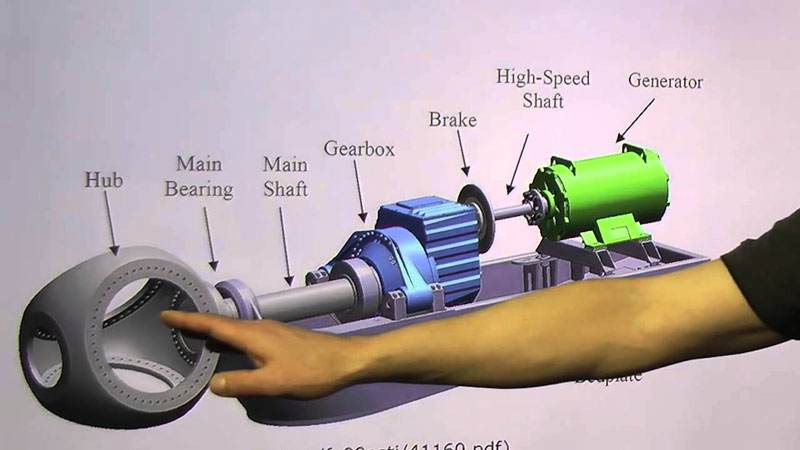# Generator Terminology###### Alternating Current (AC)

Current which varies from zero to a positive maximum to zero to a negative maximum to zero, a number of times per second, the number being expressed
in cycles per second of Hertz (HZ).

###### Alternator

A device for converting mechanical energy into electrical energy.

###### Amperage

The strength or intensity of an electric current, measured in amperes (AMPS).

###### Battery Charge Rectifier

A component which changes AC voltage from the battery charge windings (within the STATOR) to DC voltage. This voltage could b used to charge a battery.

###### Brush

A conducting element, usually graphite and/or copper, which maintains sliding electrical contact between a stationary and a moving element.

###### Conductor

A wire or cable designed for the passage of electrical current.

###### Core

The laminations in the generator constituting the magnetic structure thereof.

The metal frame that surrounds and protects the generator/engine

###### Current

The flow rate of electricity.

###### Cycle

One complete reversal of alternating current of voltage, from zero to a positive maximum to zero to a negative maximum back to zero. The number of cycles per second is the frequency, expressed in Hertz (HZ).

###### Diode

A solid state device which allows current to pass in one direction only. Since it allows only one half cycle of an alternating current pass, its output will be unidirectional and it may be considered a rectifying element.

###### Direct Current (DC)

An electric current flows in one direction only. DC is produced by chemical action (i.e. a storage battery) or by electromagnetic induction.

###### Dynamo

A machine for converting mechanical energy into electrical energy by electromagnetic induction - a generator.

###### Electro-Motive Force (EMF)

The force which causes current to flow in a conductor; in other words, the voltage potential.

###### Full Power Outlet

Enables you to draw the full power of the generator out of one outlet.

###### Generator

A general name for a device that converts mechanical energy into electrical energy. The electrical energy may be direct current (DC) or alternating current (AC).

###### Ground

A connection, intentional or accidental, between an electrical circuit and the earth or some conduction body serving in the place of the earth

###### Idle Control

A system that controls the idle speed of the engine in direct relation to the electrical load.

###### Ignition Coil

A device used to supply DC voltage to the spark plugs.

###### Magneto

An alternator with permanent magnets used to generate current for ignition in an internal combustion engine.

###### Ohm

Unit of electrical resistance. One volt will cause a current of one flow through a resistance of one ohm.

###### Phase

The uniform periodic change in amplitude or magnitude of an alternating current. Three phase alternating current consists of three different sine wave current consists of three different sine wave current flows, different in phase by 120 degrees from each other.

###### Power

Transfer System A system to safely wire your generator to your home's electrical system

###### Rated Speed

Revolutions per minute at which the set is designed to operate.

###### Rated Voltage

The rated voltage of an engine generator set is the voltage at which it is designed to operate.

###### Rear Bearing Carrier

The casting which houses the rotor bearing which supports the rotor shaft.

###### Rectifier

A device that converts AC to DC.

###### Relay

An electrically operated switch usually used in control circuits and whose contacts are considered low amperage, compared to a contactor.

###### Resistance

Opposition to the flow of current.

###### Rotor

The rotating element of a generator.

###### Single Phase

An AC load, or source of power normally having only two input terminals if a load or two output terminals if a source.

###### Stator

The stationary part of a generator.

###### Vibration Mount

A rubber device located between the engine or generator and the cradle to minimize vibration.

###### Volt

The unit of electromotive force. That electromotive force which when steadily applied to a conductor whose resistance is one ohm will produce a current of one ampere.

###### Voltage

Electrical potential difference expressed in volts.

###### Voltage Regulator

A component which automatically maintains proper generator voltage by controlling the amount of DC exitation to the rotor.

###### Watt

Unit of electrical power. In DC equals volts times amperes. In AC equals effective volts times effective amps times power factor times a consistent dependent on the number of phases. 1 kilowatt - 1,000 watts.

###### Winding

All the coils of a generator. Stator winding consists of a number stator coils and their interconnections. Rotor windings consist of all windings and connections on the rotor poles